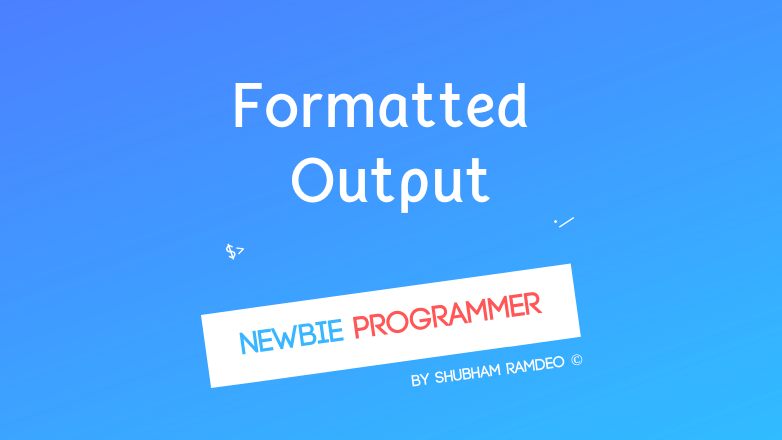July 14, 2015

# Basic Formatted Output

That’s how computers talk ! Using screen to print, formatted output !Welcome back to the Newbie Programmer Series. In the last part we have discussed how variables work. In this part we will do some basic input and output stuffs. I mean how to talk with computer ? A basic input output system is that we give data with keyboard and computer replies on the screen. We will do that here. So if you are new to this series, please go to the index and read out all the previous parts so that you can easily understand what I am talking about.

### Formatted Output using printf

For showing anything on the screen, we can use printf :

printf  ( “S_entence to be printed” , information ) ;_

For example :

``````#include <stdio.h>
main()
{
printf ("Hello there !");
}
``````

Output :

``````Hello there !
``````

If you have added some extra things :

``````...
printf("    Hello    .. There ! !  ");
...
``````

Output :

``````    Hello    .. There ! !
``````

So you see it shows up exactly whatever written in between those double commas.

Now see this one :

``````#include <stdio.h>
main()
{
printf("Hello there");
printf("My name is Shubham Ramdeo");
}
``````

Output :

``````Hello ThereMy name is Shubham Ramdeo
``````

But I want it in the next line. So I will use \n which simply indicates the computer to move to a new line. So I will modify the above code as :

``````...
printf("Hello There \n");
printf("My name is Shubham Ramdeo \n");
...
``````

Output :

``````Hello There
My name is Shubham Ramdeo
``````

There are many such things you can play with.

• \n to move to a new line.
• \t for a horizontal tab.
• \v for a vertical tab.
• \b for a backspace
• \ for \ itself !

Let me elaborate

``````#include <stdio.h>
main()
{
printf("Hello There !\nMy name is Shubham Ramdeo\n\v\tHow are you ?");
}
``````

Now if you carefully observe the use of those \n \t etc, you can see that its output is :

``````Hello There
My name is Shubhbam Ramdeo

How are you ?
``````

What’s that // ? See below example :

``````#include <stdio.h>
main()
{
printf(" A/B is division \n");
}
``````

It will not give the expected output, try it. But the correct code is ;

``````#include <stdio.h>
main()
{
printf(" A//B is division \n");
}
``````

Try to play with them, comment your cool codes below.

#### Displaying Values

As in the last part, we have seen that in the printf, you can use :

• %d for printing integers
• %f for printing fractions
• %c for printing characters
• %% for % itself

Here printing means to print on the screen.

For example :

``````#include <stdio.h>
main()
{
int a = 20;
int b = 40;
int c = a + b;
printf("Sum is %d \n", c);
}
``````

Output :

``````Sum is 60
``````

Basically, these %d, %f, %c get replaced by the values written after the comma. Here %c is replaced by the value of variable c.

You can do such things in a simpler way too :

``````#include <stdio.h>
main()
{
int a = 20, b = 40;
printf("Sum is %d \n", a + b);
}
``````

In both the above versions, changing the values of variables will also change the output. If I made a = 5 and b = 10, I will get “Sum is 15”.

This one is little direct, without involving any variables :

``````#include <stdio.h>
main()
{
printf("Sum is %d", 20 + 40 );
}
``````

If you want to add fractions, use float instead of int and use %f instead of %d.

This is a little horrible :

``````#include <stdio.h>
main()
{
int class = 10;
float perc = 98.8;
printf("\tShubham Ramdeo\nI am in class %dth\nI got %f%%\n%c Grade.\n", class, perc, grade);
}
``````

How will it work ?

• Firstly the three variables are created in the computer memory. Then the printing starts on the screen.
• The first \t takes a tab space, and prints “Shubham Ramdeo”.
• The it comes to a new line because of \n.
• Then it prints “I am in class %dth”, and replace %d with the corresponding variable class which is 10, so the final printing is “I am in class 10th”.
• Then it again comes to a new line and prints “I got %f%%”. Here %% is replaced by a single % and %f is replaced by the corresponding variable perc which is 98.8 so final printing is “I got 98.80%”.
• Then it again comes to new line and prints “%c Grade” And here %c is replaced by the corresponding variable grade which is ‘A’ so final printing is “A Grade”

The overall Output is :

``````         Shubham Ramdeo
I am in 10th Class
I got 98.80%
``````

The fun part is that changing the variable values will change the output too :

``````#include <stdio.h>
main()
{
int class = 9;
float perc = 94;
printf("\tShubham Ramdeo\nI am in class %dth\nI got %f%%\n%c Grade.\n", class, perc, grade);
}
``````

Will say :

``````        Shubham Ramdeo
I am in 9th Class
I got 94.00%
``````

You see why variables are handy.

This part has gone big enough ! We have learn how to say, now we will discuss how to hear ! In the next part we will discuss how to take input from the keyboard. So Stay connected ! Thanks for reading. :)

(prev)
(next)